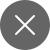# Backpropagation Detailed Explanation

7+
##### This paper describes the working of Backpropagation and its importance and is much faster than several neural networks. Using Backpropagation, we can use neural nets to solve the previously unsolvable problems.

Backpropagation was introduced in the early ’70s but it got appreciation through a research paper in 1980. Backpropagation is the fundamental block to many other Neural network algorithms and at present, it is the workhorse of training in Deep learning.

Backward propagation of errors is in short known as Backpropagation. We use the backpropagation algorithm widely to train the neural networks. This is a supervised learning algorithm of Artificial neural networks that work with the help of Gradient descent.

In the above picture, we can see the path that formed by connecting the dots which leads to the steepest descent from the starting. Here the starting point can be any point on the graph. And the steepest descent in the graph is the area in blue color indicated by red color arrows.

### Backpropagation

Backpropagation is a supervised learning algorithm that considers an error function and an ANN. It calculates the gradient of the respective error function concerning the weights in the neural networks. We use backpropagation to train a model through a Chain rule method.

The name backpropagation itself explains that there will be the transmission of data in the backward direction. After each forward transmission there occurs a backpropagation which performs the backward pass by adjusting the weights accordingly.

In this article, I will walk you through the process of Backpropagation and applications of Backpropagation using a three-layer neural network. Let’s delve in and know more about backpropagation in Deep learning.

## Working of Backpropagation

To know how the Backpropagation algorithm works? We will consider a simple neural network with three layers, one input layer, one hidden layer, and one output layer.

In the above picture i1, i2 are input neurons, and h1, h2 are hidden neurons, and o1, o2 are output neurons. B1 and B2 are biased. All the w’s represent the weights of connections between the neurons in the different layers. The input we feed into the neurons in the input layer can be scalar, feature vector, multidimensional matrices.

We feed the input to the input layer and the cumulative output from the input layer goes through the hidden layer as input. The cumulative output of the hidden layer goes through the output layer as input. Then we get the final output through the output layer. Now, we will backpropagate the error calculated at the output layer to the input layer back and we will update the weights accordingly.

Now, let’s see how to calculate the output of the input layer in this current network. These outputs are the inputs to hidden layers that are h1 and h2.

h1 = i1w1 + i2w2 + b1

h2 = i1w3 + i2w4 + b1

Before going further let’s take a minute and know about the activation function

## Activation Function

A mathematical equation that helps to determine the output in a neural network is an Activation function. We connect this activation function to each node in a network. The activation of this function depends directly on the input. In an activation function, a non-linear transformation occurs on the input given and this function makes the model capable to learn. So that we can apply it to more advanced problems.

There are several types of activation functions that we can use in Deep learning. A few among them are Sigmoid, relu, tanh, Softmax, etc.

Now, we will apply the activation function to get the output at each node in the middle of the network (output layers and hidden layers). One of the activation functions is sigmoid. The equation below represents the sigmoid function. Using the sigmoid function is very normal in the case of output layers.

With the help of the above equation, we find the outputs of the nodes h1 and h2. Similarly, we find the final output of the network with the help of the activation function. The variable below aj is the output of the layer ‘l’, and wjk is the weight of the connection between node j and node k in the layer l. This is the generalized formula to find the output of a particular layer ‘l’.

After finding the values o1 and o2 in the above network we calculate the error. Here we find the difference between the target value (t) and predicted value (p). We calculate this error using a cost function. This cost function can be Mean square error (MSE), Cross-Entropy, etc. In our case, we consider the mean square error function as our cost function. The equation below calculates the error in our network. Yi is the target value and y’i is the predicted value.

Based on the error MSE value we adjust the weights of the connections so that our predicted value is much closer to the target value. Now, we will backpropagate these errors by adjusting the weights.

### Backward Pass

The main of backpropagation is to minimize the cost function with the help of adjustments in the connections between nodes and weights concerning those connections. Gradient descent helps us to adjust the weights. Let’s assume C(x1, x2, x3, …….) is a vector and the gradient function of this vector is shown below which is nothing but the partial derivative of C concerning x. This gradient helps us know how much the variable x should be changed such that C will be minimum.

With the help of the chain rule in differentiation, we will compute the gradient. This means we calculate the error at each node within the hidden layers and use those values to calculate the gradient. First, we will backpropagate from the output layer to the hidden layer than from the hidden layer to the input layer.

## Conclusion